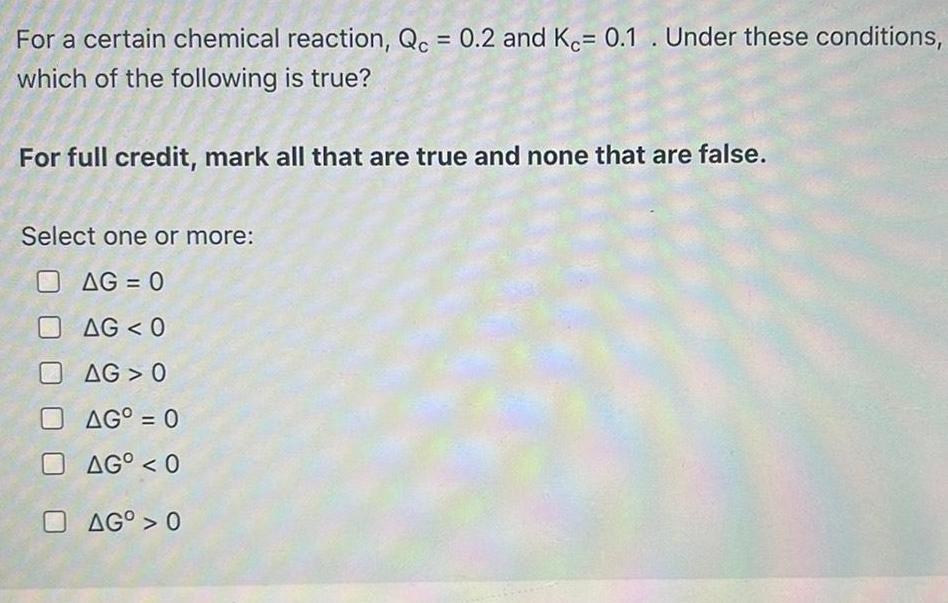Physical Chemistry
Equilibrium
For a certain chemical reaction, Qc = 0.2 and Kc= 0.1. Under these conditions, which of the following is true? For full credit, mark all that are true and none that are false. Select one or more: ΔG < 0 ΔG 0 ΔG° = 0 ΔGO < 0 ΔG° > 0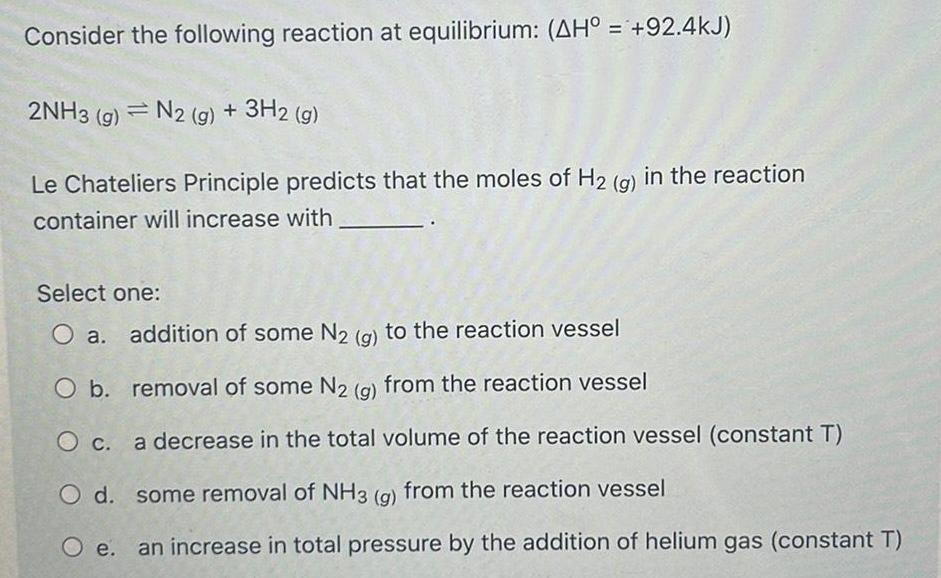Physical Chemistry
Equilibrium
Consider the following reaction at equilibrium: (ΔH° = +92.4kJ) 2NH3 (g) = N2 (g) + 3H2 (g) Le Chateliers Principle predicts that the moles of H2 (g) in the reaction container will increase with Select one: O a. addition of some N₂ (g) to the reaction vessel O b. removal of some N2 (g) from the reaction vessel O c. a decrease in the total volume of the reaction vessel (constant T) O d. some removal of NH3 (g) from the reaction vessel O e. an increase in total pressure by the addition of helium gas (constant T)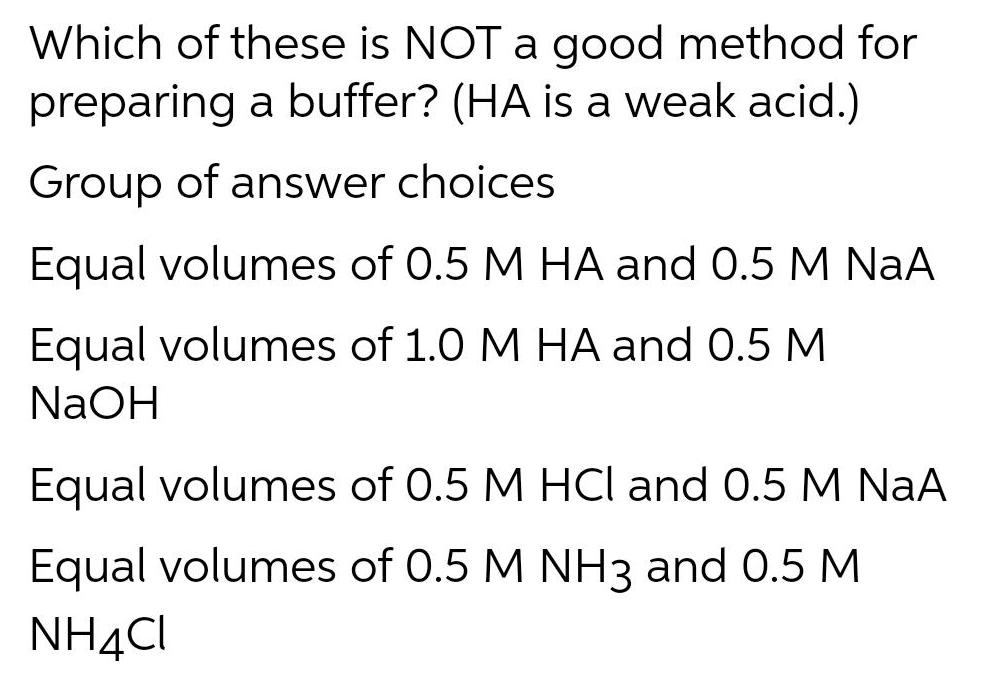Physical Chemistry
Equilibrium
Which of these is NOT a good method for preparing a buffer? (HA is a weak acid.) Group of answer choices Equal volumes of 0.5 M HA and 0.5 M NaA Equal volumes of 1.0 M HA and 0.5 M NaOH Equal volumes of 0.5 M HCl and 0.5 M NaA Equal volumes of 0.5 M NH3 and 0.5 M NH4Cl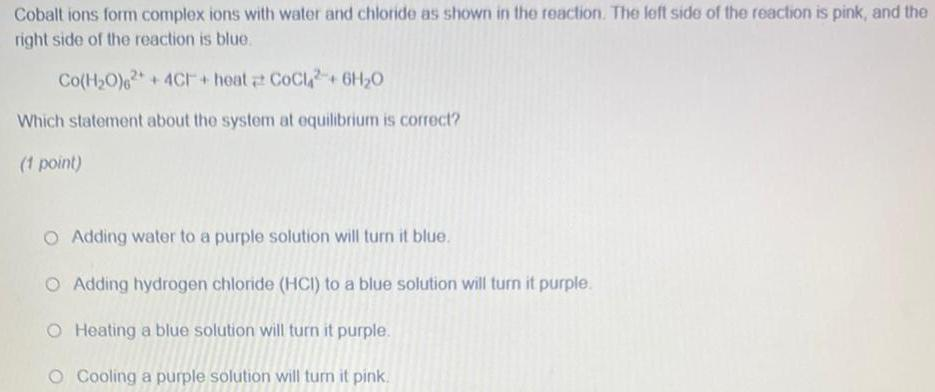Physical Chemistry
Equilibrium
Cobalt ions form complex ions with water and chloride as shown in the reaction. The left side of the reaction is pink, and the right side of the reaction is blue. Co(H₂O)2 + 4C+ heat <--> CoCl2 + 6H₂O Which statement about the system at equilibrium is correct? Adding water to a purple solution will turn it blue. Adding hydrogen chloride (HCI) to a blue solution will turn it purple. Heating a blue solution will turn it purple. Cooling a purple solution will turn it pink.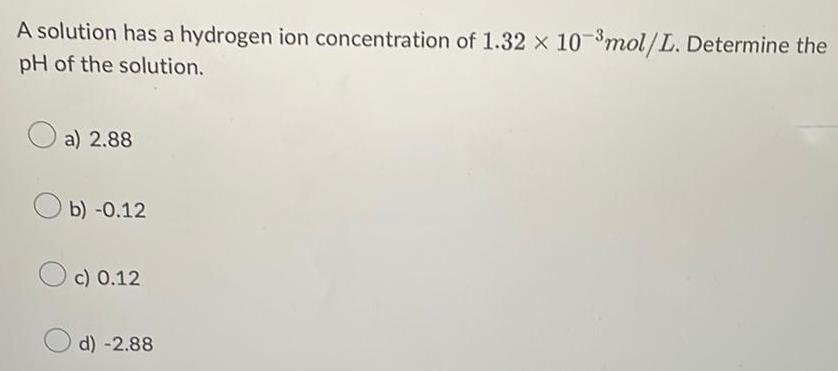Physical Chemistry
Equilibrium
A solution has a hydrogen ion concentration of 1.32 x 10-³ mol/L. Determine the pH of the solution. a) 2.88 b) -0.12 c) 0.12 d) -2.88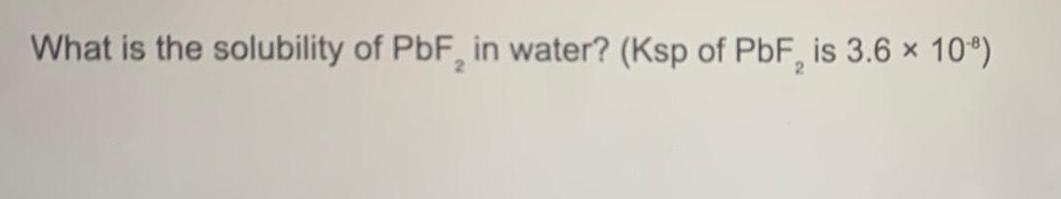Physical Chemistry
Equilibrium
What is the solubility of PbF2 in water? (Ksp of PbF2 is 3.6 × 10-8)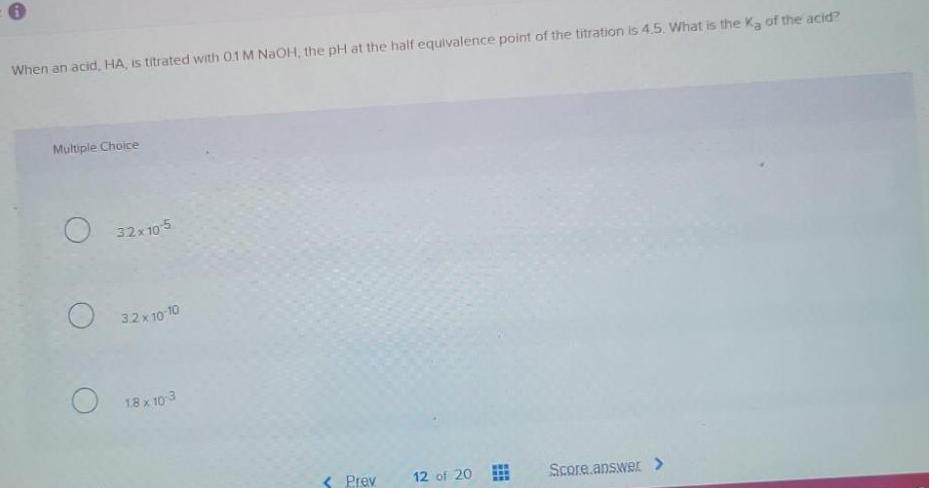Physical Chemistry
Equilibrium
When an acid, HA, is titrated with 0.1 M NaOH, the pH at the half equivalence point of the titration is 4.5. What is the Ka of the acid? Multiple Choice 32x10-5 3.2x10-10 1.8 x 10-3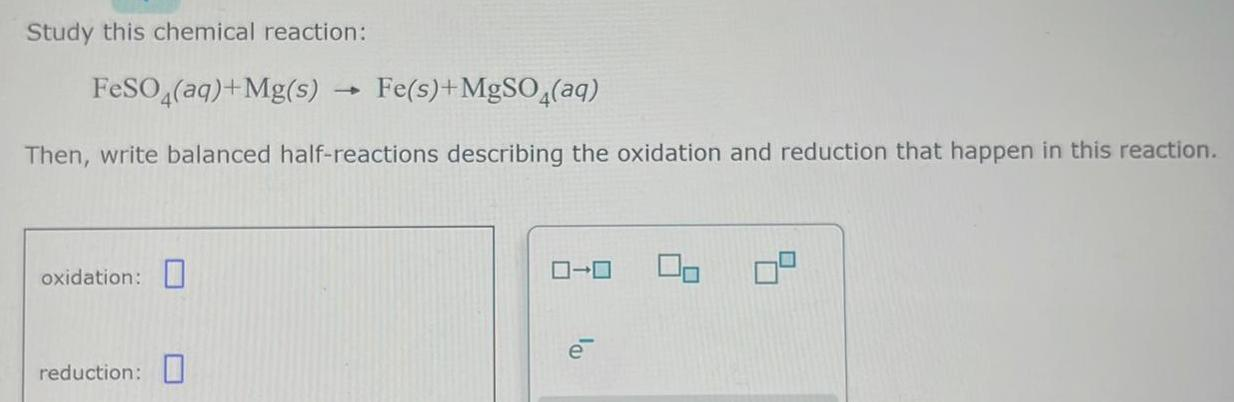Physical Chemistry
Equilibrium
Study this chemical reaction: FeSO4(aq) + Mg(s) Fe(s)+MgSO4(aq) Then, write balanced half-reactions describing the oxidation and reduction that happen in this reaction. oxidation: reduction: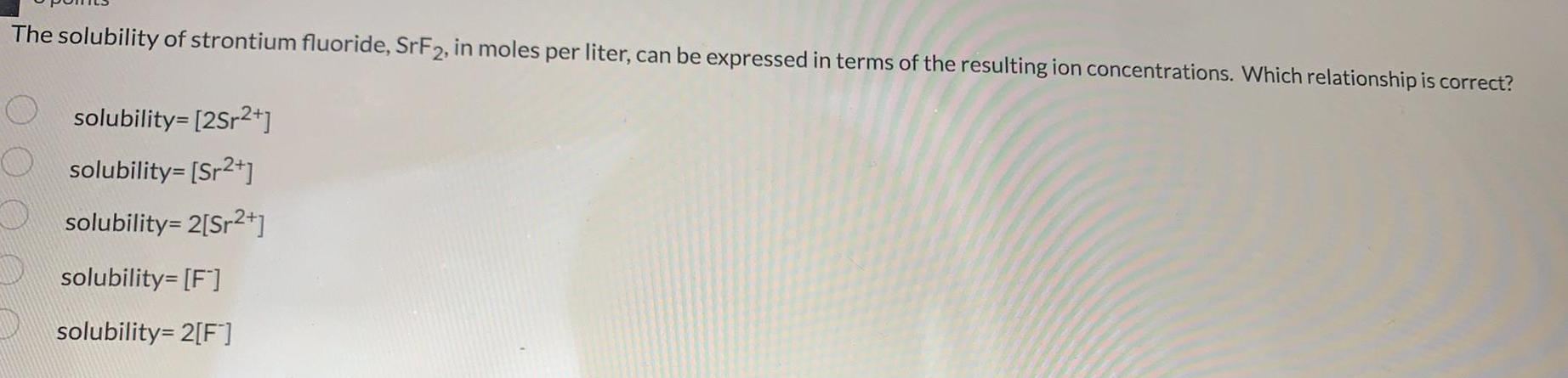Physical Chemistry
Equilibrium
The solubility of strontium fluoride, SrF2, in moles per liter, can be expressed in terms of the resulting ion concentrations. Which relationship is correct? solubility=[2Sr2+] solubility=[Sr2+] solubility= 2[Sr2+] solubility=[F-] solubility= 2[F-]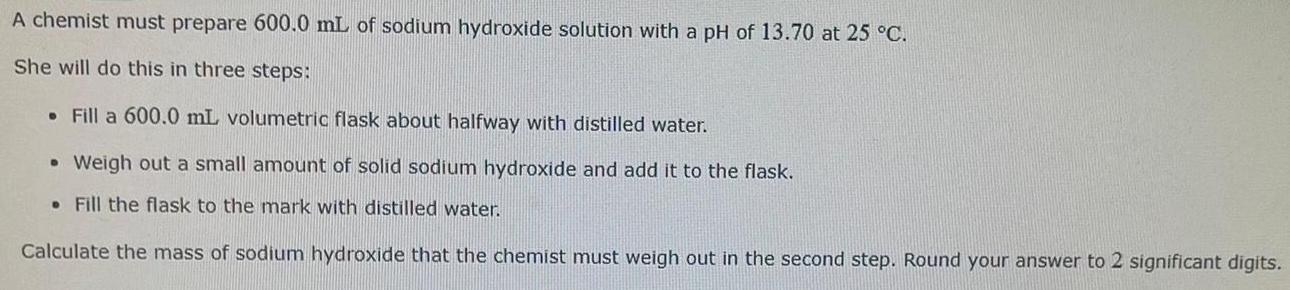Physical Chemistry
Equilibrium
A chemist must prepare 600.0 mL of sodium hydroxide solution with a pH of 13.70 at 25 °C. She will do this in three steps: Fill a 600.0 mL volumetric flask about halfway with distilled water. Weigh out a small amount of solid sodium hydroxide and add it to the flask. Fill the flask to the mark with distilled water. Calculate the mass of sodium hydroxide that the chemist must weigh out in the second step. Round your answer to 2 significant digits.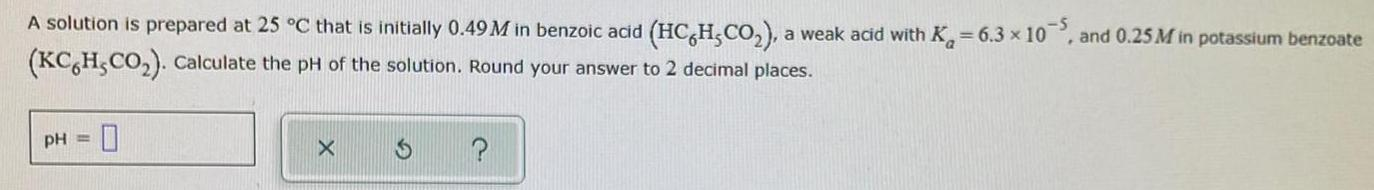Physical Chemistry
Equilibrium
A solution is prepared at 25 °C that is initially 0.49 M in benzoic acid (HCH,CO₂), a weak acid with K = 6.3 x 10, and 0.25M in potassium benzoate (KC6H5CO₂). Calculate the pH of the solution. Round your answer to 2 decimal places.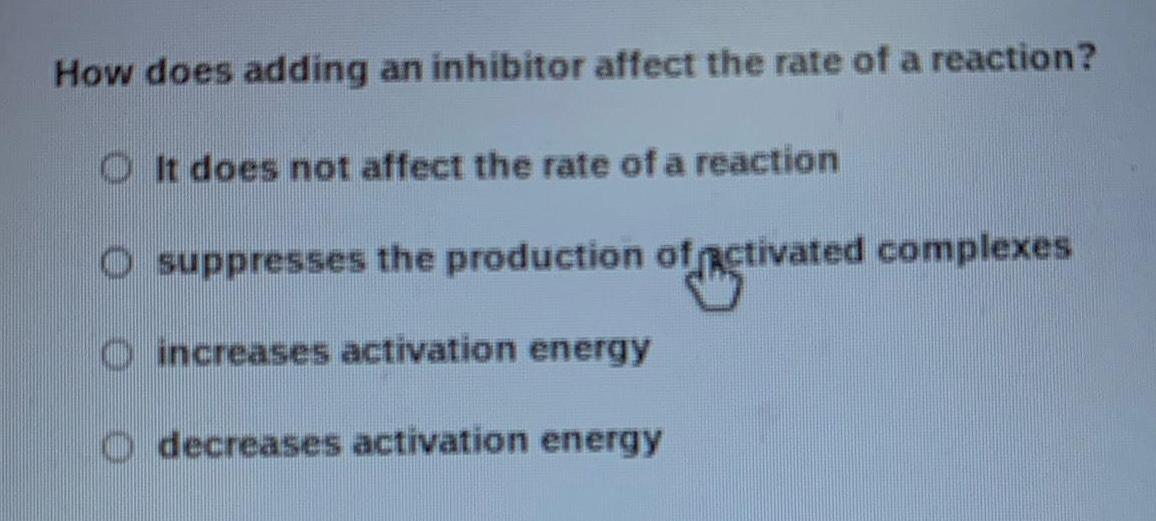Physical Chemistry
Equilibrium
How does adding an inhibitor affect the rate of a reaction? It does not affect the rate of a reaction suppresses the production of activated complexes increases activation energy decreases activation energy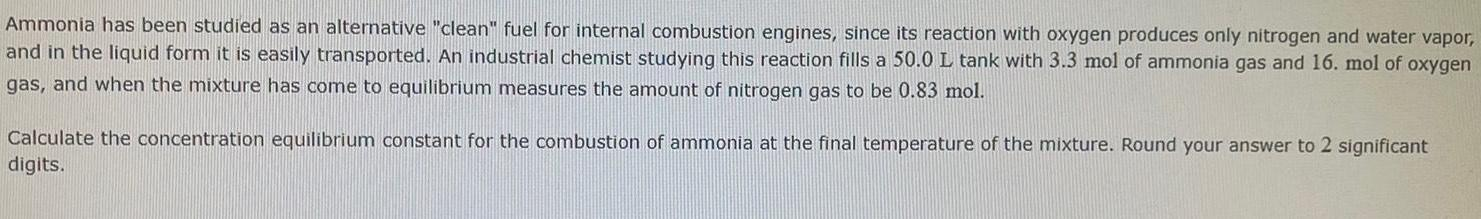Physical Chemistry
Equilibrium
Ammonia has been studied as an alternative "clean" fuel for internal combustion engines, since its reaction with oxygen produces only nitrogen and water vapor, and in the liquid form it is easily transported. An industrial chemist studying this reaction fills a 50.0 L tank with 3.3 mol of ammonia gas and 16. mol of oxygen gas, and when the mixture has come to equilibrium measures the amount of nitrogen gas to be 0.83 mol. Calculate the concentration equilibrium constant for the combustion of ammonia at the final temperature of the mixture. Round your answer to 2 significant digits.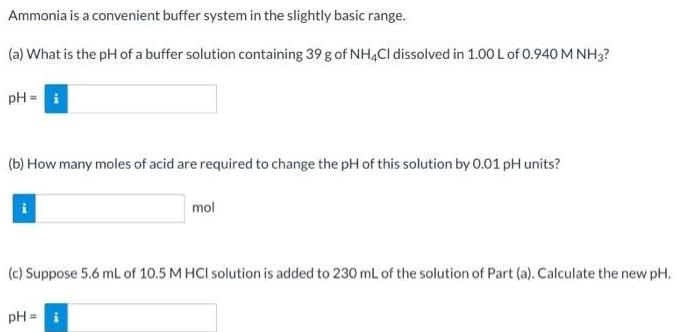Physical Chemistry
Equilibrium
Ammonia is a convenient buffer system in the slightly basic range. (a) What is the pH of a buffer solution containing 39 g of NH4Cl dissolved in 1.00 L of 0.940 M NH3? pH = (b) How many moles of acid are required to change the pH of this solution by 0.01 pH units? mol (c) Suppose 5.6 mL of 10.5 M HCI solution is added to 230 mL of the solution of Part (a). Calculate the new pH. pH=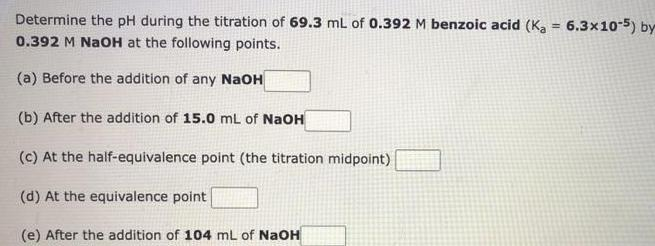Physical Chemistry
Equilibrium
Determine the pH during the titration of 69.3 mL of 0.392 M benzoic acid (Ka = 6.3x10-5) by 0.392 M NaOH at the following points. (a) Before the addition of any NaOH (b) After the addition of 15.0 mL of NaOH (c) At the half-equivalence point (the titration midpoint) (d) At the equivalence point (e) After the addition of 104 mL of NaOHPhysical Chemistry
Equilibrium
Write the net ionic equation for the neutralization of nitric acid by sodium hydroxide in aqueous solution. H+(aq) + OH¨(aq) → H₂O(l) H+(aq)+NO3(aq) +OH-(aq)→ NO3(aq)+ H₂O(l) HNO3(aq) +Na+(aq)+ OH-(aq)→ Na+(aq) +NO3(aq) +H₂O(l) HNO3(aq) +OH-(aq)→ NO3-(aq) + H₂O(l)